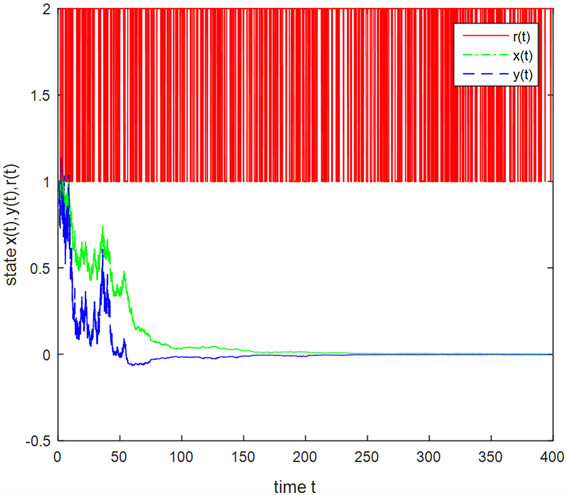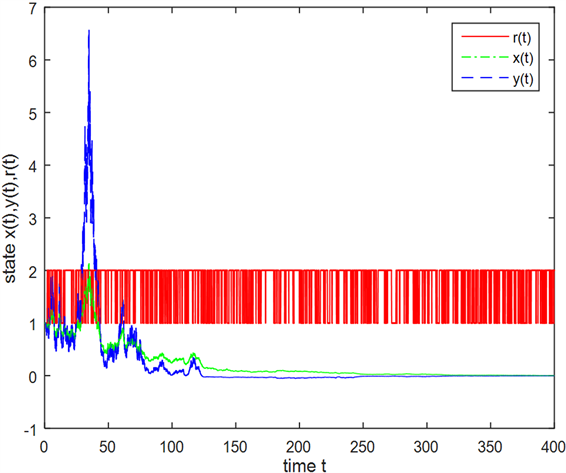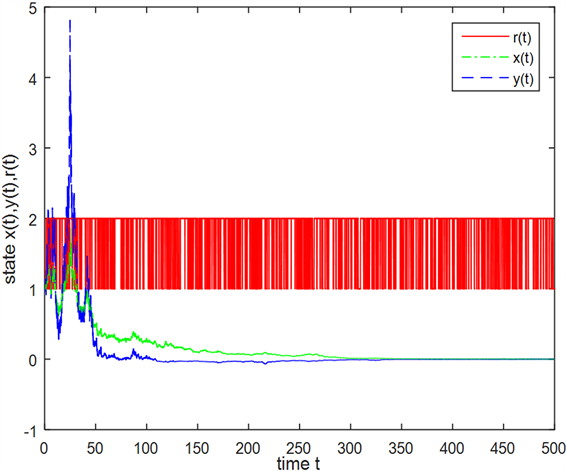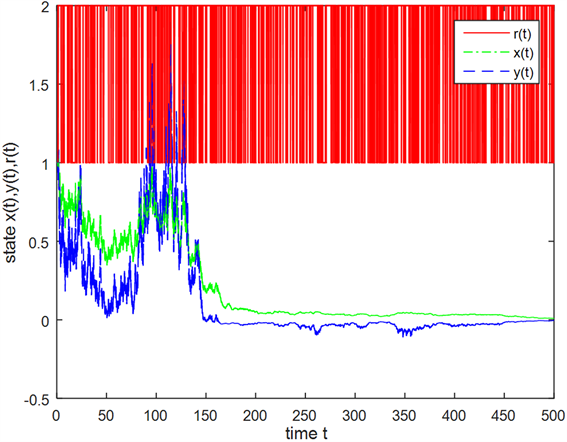﻿ 马尔可夫调制的随机时滞IS-LM模型的随机稳定性仿真

# 马尔可夫调制的随机时滞IS-LM模型的随机稳定性仿真Simulation of Stochastic Stability of Delayed IS-LM Model with Markovian Switching

Abstract: This paper studies that the economic system is in the random environment which has delayed time in taxation and Markov chain with two discrete values. By using the method of stochastic stability and the given parameters, this paper analyzes the stability and simulation of the solution of the standard random IS-LM model with Markovian Switching and delayed time.

1. 引言

2. 预备知识及模型构建

Mao (2001)研究了如下的马尔可夫调制的随机微分方程  ：

$\text{d}x\left(t\right)=f\left(x\left(t\right),x\left(t-\tau \right),t,r\left(t\right)\right)\text{d}t+g\left(x\left(t\right),x\left(t-\tau \right),t,r\left(t\right)\right)\text{d}w\left( t \right)$

$P\left\{r\left(t+\Delta \right)=j|r\left(t\right)=i\right\}=\left\{\begin{array}{l}{\gamma }_{ij}\Delta +o\left(\Delta \right),\\ 1+{\gamma }_{ij}\Delta +o\left(\Delta \right),\end{array}$ $\begin{array}{l}i\ne j\\ i=j\end{array}$

V. Torre (1977)用分岔理论分析了非均衡市场的完全凯恩斯系统 

$\left\{\begin{array}{l}\stackrel{˙}{Y}\left(t\right)=\alpha \left(I\left(Y\left(t\right),R\left(t\right)\right)-S\left(Y\left(t\right),R\left(t\right)\right)\right),\\ \stackrel{˙}{R}\left(t\right)={\beta }_{0}\left(L\left(Y\left(t\right),R\left(t\right)\right)-{L}_{s}\right),\end{array}$

$\left\{\begin{array}{l}\stackrel{˙}{Y}\left(t\right)=\alpha \left(\left(\sigma +\gamma -1\right)Y\left(t\right)-\sigma \epsilon Y\left(t-\tau \right)-{\beta }_{1}R\left(t\right)+G\right),\\ \stackrel{˙}{R}\left(t\right)=\beta {}_{0}\left(uY\left(t\right)-{\beta }_{2}R\left(t\right)-{L}_{s}\right)\end{array}$ (1)

$\left({Y}^{*},{R}^{*}\right)=\left(\frac{{\beta }_{2}G+{\beta }_{1}{L}_{s}}{u{\beta }_{1}-{\beta }_{2}\left(\sigma \left(1-\epsilon \right)-1+\gamma \right)},\frac{uG+\left(\sigma \left(1-\epsilon \right)-1+\gamma \right){L}_{s}}{u{\beta }_{1}-{\beta }_{2}\left(\sigma \left(1-\epsilon \right)-1+\gamma \right)}\right)$

$x\left(t\right)=Y\left(t\right)-{Y}^{*},x\left(t-\tau \right)=Y\left(t-\tau \right)-{Y}^{*}$$y\left(t\right)=R\left(t\right)-{R}^{*}$ ，则模型(1)变成

$\left\{\begin{array}{l}\stackrel{˙}{x}\left(t\right)=\alpha \left(\left(\sigma +\gamma -1\right)x\left(t\right)-\sigma \epsilon x\left(t-\tau \right)-{\beta }_{1}y\left(t\right)\right),\\ \stackrel{˙}{y}\left(t\right)={\beta }_{0}\left(ux\left(t\right)-{\beta }_{2}y\left(t\right)\right)\end{array}$ (2)

$\left\{\begin{array}{l}\stackrel{˙}{x}\left(t\right)=\left(\alpha \left(\sigma +\gamma -1\right)+{\sigma }_{1}\omega \left(t\right)\right)x\left(t\right)+\left(-\alpha \sigma \epsilon +{\sigma }_{2}\omega \left(t\right)\right)x\left(t-\tau \right)+\left(-\alpha {\beta }_{1}+{\sigma }_{3}w\left(t\right)\right)y\left(t\right),\\ \stackrel{˙}{y}\left(t\right)=\left(\left({\beta }_{0}u+{\sigma }_{4}\omega \left(t\right)\right)x\left(t\right)+\left(-{\beta }_{0}{\beta }_{2}+{\sigma }_{5}w\left(t\right)\right)y\left(t\right)\right)\end{array}$ (3)

$\text{d}X\left(t\right)=\left(AX\left(t\right)+BX\left(t-\tau \right)\right)dt+\left(CX\left(t\right)+DX\left(t-\tau \right)\right)\text{d}w\left(t\right)$ (4)

$A=\left(\begin{array}{cc}\alpha \left(\sigma +\gamma -1\right)& -\alpha {\beta }_{1}\\ {\beta }_{0}u& -{\beta }_{0}{\beta }_{2}\end{array}\right),B=\left(\begin{array}{cc}-\alpha \sigma \epsilon & 0\\ 0& 0\end{array}\right)$$C=\left(\begin{array}{cc}{\sigma }_{1}& {\sigma }_{3}\\ {\sigma }_{4}& {\sigma }_{5}\end{array}\right),D=\left(\begin{array}{cc}{\sigma }_{2}& 0\\ 0& 0\end{array}\right)$

$dX\left(t\right)=\left(A\left(r\left(t\right)\right)X\left(t\right)+B\left(r\left(t\right)\right)X\left(t-\tau \right)\right)\text{d}t+\left(C\left(r\left(t\right)\right)X\left(t\right)+D\left(r\left(t\right)\right)X\left(t-\tau \right)\right)\text{d}w\left(t\right)$ (5)

3. 案例仿真

${\alpha }_{1}=0.011,{\sigma }_{1}=0.018,{\gamma }_{1}=0.049,{\beta }_{01}=0.024,{\beta }_{11}=0.056,{\beta }_{21}=0.035,{u}_{1}=0.046$

${\epsilon }_{1}=0.021,{\sigma }_{11}=0.048,{\sigma }_{21}=0.024,{\sigma }_{31}=0.015,{\sigma }_{41}=0.071,{\sigma }_{51}=0.171$

${\alpha }_{2}=0.011,{\sigma }_{2}=0.016,{\gamma }_{2}=0.029,{\beta }_{02}=0.025,{\beta }_{12}=0.065,{\beta }_{22}=0.065,{u}_{2}=0.026$

${\epsilon }_{2}=0.031,{\sigma }_{12}=0.058,{\sigma }_{22}=0.016,{\sigma }_{32}=0.025,{\sigma }_{42}=0.061,{\sigma }_{52}=0.271$Figure 1. Solution x(t), y(t) of sde and r(t) (time lag = 0.2)Figure 2. Solution x(t), y(t) of sde and r(t) (time lag = 0.2)Figure 3. Solution x(t), y(t) of sde and r(t) (time lag = 0.8)Figure 4. Solution x(t), y(t) of sde and r(t) (time lag = 0.8)

 Mao, X., Matasov, A. and Piunovskiy, A.B. (2000) Stochastic Differential Delay Equations with Markovian Switching. Bernoulli, 1, 73-90.
https://doi.org/10.2307/3318634

 Torre, V. (1977) The Existence of Limit Cycles and Control in Complete Keynesian Systems by Theory of Bifurcations. Econometrica, 45, 1457-1466.
https://doi.org/10.2307/1912311

 Turnovsky, S.J. (1996) Methods of Macroeconomic Dynamics. MIT Press, Boston.

 Bing, L., Dingshi, L. and Daoyi, X. (2013) Stability Analysis for Impulsive Stochastic Delay Differential Equations with Markovian Switching. Journal of the Franklin Institute, 350, 1848-1864.

Top